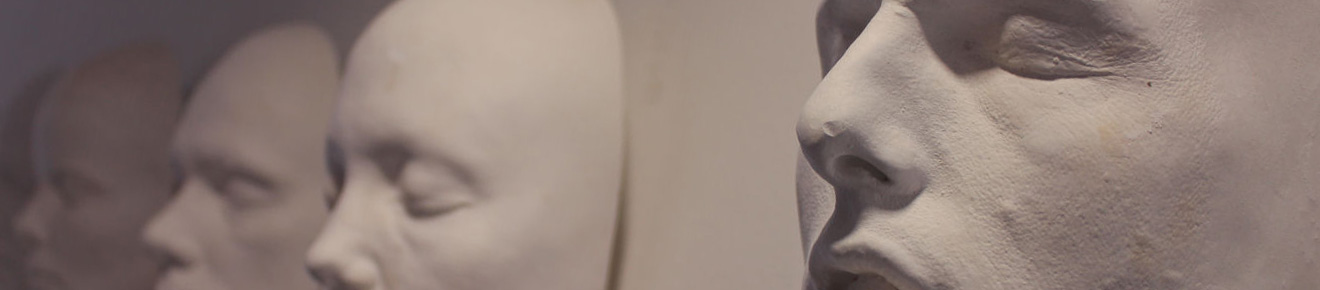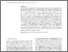# Electromagnetic Counterparts to Structured Jets from Gravitational Wave Detected Mergers

Lamb, GP and Kobayashi, S (2017) Electromagnetic Counterparts to Structured Jets from Gravitational Wave Detected Mergers. Monthly Notices of the Royal Astronomical Society, 472 (4). pp. 4953-4964. ISSN 0035-8711Preview
Text
Electromagnetic_Counterparts_to_Structured_Jets_from_from_Gravitational_Wave_Detected_Mergers.pdf - Published Version

We show the peak magnitude for orphan afterglows from the jets of gravitational wave (GW) detected black-hole/neutron star - neutron star (BH/NS-NS) mergers highly depends on the jet half-opening angle $\theta_j$. Short $\gamma$-ray bursts (GRB) with a homogeneous jet structure and $\theta_j>10^\circ$, the orphan afterglow viewed at the typical inclination for a GW detected event, 38$^\circ$, is brighter at optical frequencies than the comparable macronova emission. Structured jets, where the energetics and Lorentz factor $\Gamma$ vary with angle from the central axis, may have low-$\Gamma$ components where the prompt emission is suppressed; GW electromagnetic (EM) counterparts may reveal a population of failed-GRB orphan afterglows. Using a Monte Carlo method assuming a NS-NS detection limit we show the fraction of GW-EM counterparts from homogeneous, two-component, power-law structured, and Gaussian jets where the variable structure models include a wide low energy and $\Gamma$ component: for homogeneous jets, with a {$\theta_j=6^\circ$ and typical short GRB parameters, we find {\it r}-band magnitude $m_r\leq21$ counterparts for $\sim 13.6\%$ of GW detected mergers; where jet structure extends to a half-opening angle of $25^\circ$, two-component jets produce $m_r\leq21$ counterparts in $\sim30\%$ of GW detected mergers; power-law structured jets result in $\sim37\%$; and Gaussian jets with our parameters $\sim13\%$.} We show the features in the lightcurves from orphan afterglows can be used to indicate the presence of extended structure.View Item